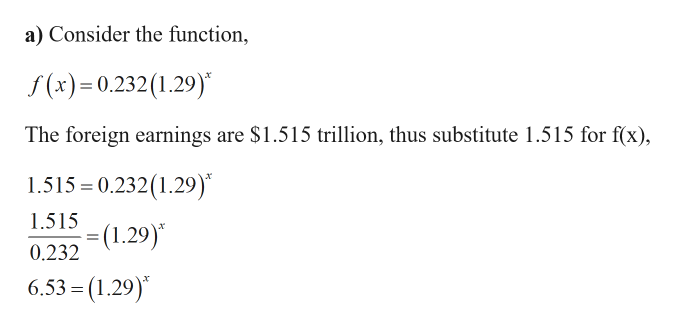# The amount of foreign earnings​ (in trillions of​ dollars) kept overseas can be approximated by the functionf(x)=0.232(1.29)^x​wherex=5corresponds to the year 2005. Find the first full year in which the foreign earnings kept overseas exceeds the following amounts. ​(a)  ​\$1.51.5trillion​(b)​ \$2.62.6trillion

Question
9 views
The amount of foreign earnings​ (in trillions of​ dollars) kept overseas can be approximated by the function
f(x)=0.232(1.29)^x​
where
x=5
corresponds to the year 2005. Find the first full year in which the foreign earnings kept overseas exceeds the following amounts.

​(a)
​\$1.51.5
trillion
​(b)
​ \$2.62.6
trillion
check_circle

Step 1

Given:

Step 2

Calculation:help_outlineImage Transcriptionclosea) Consider the function, f(x)=0.232(1.29) The foreign earnings are \$1.515 trillion, thus substitute 1.515 for f(x) 1.515 0.232(1.29) 1.515 (1.29) 0.232 6.53 = (1.29) fullscreen
Step 3

Taking ln on bot...

### Want to see the full answer?

See Solution

#### Want to see this answer and more?

Solutions are written by subject experts who are available 24/7. Questions are typically answered within 1 hour.*

See Solution
*Response times may vary by subject and question.
Tagged in

### Algebra